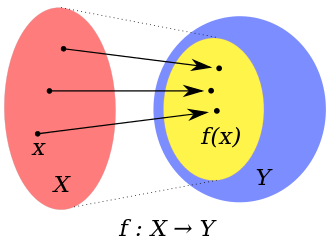# CodomainA function f from X to Y. The large blue oval is Y which is the codomain of f . The smaller (yellow) oval inside Y is the image of f .

In mathematics, the codomain or target set of a function is the set Y into which all of the output of the function is constrained to fall. It is the set Y in the notation f: XY. The codomain is also sometimes referred to as the range but that term is ambiguous as it may also refer to the image.

The codomain is part of a function f if it is defined as described in 1954 by Nicolas Bourbaki, namely a triple (X, Y, F), with F a functional subset of the Cartesian product X × Y and X is the set of first components of the pairs in F (the domain). The set F is called the graph of the function. The set of all elements of the form f(x), where x ranges over the elements of the domain X, is called the image of f. In general, the image of a function is a subset of its codomain. Thus, it may not coincide with its codomain. Namely, a function that is not surjective has elements y in its codomain for which the equation f(x) = y does not have a solution.

An alternative definition of function by Bourbaki [Bourbaki, op. cit., p. 77], namely as just a functional graph, does not include a codomain and is also widely used. For example in set theory it is desirable to permit the domain of a function to be a proper class X, in which case there is formally no such thing as a triple (X, Y, F). With such a definition functions do not have a codomain, although some authors still use it informally after introducing a function in the form f: XY.

## Examples

For a functiondefined by, or equivalently,

the codomain of f is, but f does not map to any negative number. Thus the image of f is the set; i.e., the interval [0, ∞).

An alternative function g is defined thus:While f and g map a given x to the same number, they are not, in this view, the same function because they have different codomains. A third function h can be defined to demonstrate why:The domain of h must be defined to be:.

The compositions are denoted,.

On inspection, hf is not useful. It is true, unless defined otherwise, that the image of f is not known; it is only known that it is a subset of. For this reason, it is possible that h, when composed with f, might receive an argument for which no output is defined – negative numbers are not elements of the domain of h, which is the square root function.

Function composition therefore is a useful notion only when the codomain of the function on the right side of a composition (not its image, which is a consequence of the function and could be unknown at the level of the composition) is the same as the domain of the function on the left side.

The codomain affects whether a function is a surjection, in that the function is surjective if and only if its codomain equals its image. In the example, g is a surjection while f is not. The codomain does not affect whether a function is an injection.

A second example of the difference between codomain and image is demonstrated by the linear transformations between two vector spaces – in particular, all the linear transformations fromto itself, which can be represented by the 2×2 matrices with real coefficients. Each matrix represents a map with the domainand codomain. However, the image is uncertain. Some transformations may have image equal to the whole codomain (in this case the matrices with rank 2) but many do not, instead mapping into some smaller subspace (the matrices with rank 1 or 0). Take for example the matrix T given bywhich represents a linear transformation that maps the point (x, y) to (x, x). The point (2, 3) is not in the image of T, but is still in the codomain since linear transformations fromtoare of explicit relevance. Just like all 2×2 matrices, T represents a member of that set. Examining the differences between the image and codomain can often be useful for discovering properties of the function in question. For example, it can be concluded that T does not have full rank since its image is smaller than the whole codomain.

## Notes

1. N.Bourbaki (1954). Elements de Mathematique,Theorie des Ensembles. Hermann & cie. p. 76.
2. A set of pairs is functional iff no two distinct pairs have the same first component [Bourbaki, op. cit., p. 76]
3. Mac Lane, in Scott & Jech 1967, page 232

## References

• Eccles, Peter J. (1997), An Introduction to Mathematical Reasoning: Numbers, Sets, and Functions, Cambridge University Press, ISBN 978-0-521-59718-0
• Forster, Thomas (2003), Logic, Induction and Sets, Cambridge University Press, ISBN 978-0-521-53361-4
• Mac Lane, Saunders (1998), Categories for the working mathematician (2nd ed.), Springer, ISBN 978-0-387-98403-2
• Scott, Dana S.; Jech, Thomas J. (1967), Axiomatic set theory, Symposium in Pure Mathematics, American Mathematical Society, ISBN 978-0-8218-0245-8
• Sharma, A.K. (2004), Introduction To Set Theory, Discovery Publishing House, ISBN 978-81-7141-877-0
• Stewart, Ian; Tall, David Orme (1977), The foundations of mathematics, Oxford University Press, ISBN 978-0-19-853165-4